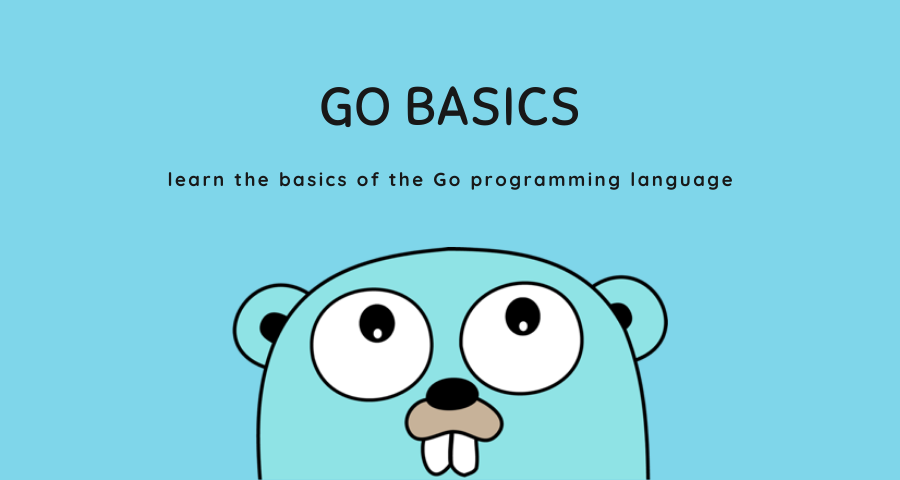# Go Basics - 3. Primitives in GoIn this article, we are going to explore some of the primitives of the Go programming language.

## Bool type

Booleans are in just about every single programming language, and Go is no exception. They represent the value of either `true` or `false`.

``````package main

import (
"fmt"
)

func main() {
var b bool = true
fmt.Printf("%v, %T", b, b)
}

/*
The result of the code above is:
true, bool
*/
``````
``````package main

import (
"fmt"
)

func main() {
var b bool = false
fmt.Printf("%v, %T", b, b)
}

/*
The result of the code above is:
false, bool
*/
``````

An interesting thing to note is that booleans are given a `zero` value when they are initialized. This means that the `bool` type is given the value of `false` unless otherwise specified.

``````package main

import (
"fmt"
)

func main() {
var b bool
fmt.Printf("%v, %T", b, b)
}

/*
The result of the code above is:
false, bool
*/
``````

## Numeric Types

Golang has several different numeric types. The first one we are going to learn about is the `int` type.

``````package main

import (
"fmt"
)

func main() {
i := 14
fmt.Printf("%v, %T", i, i)
}

/*
The result of the code above is:
14, int
*/
``````

There are 4 different `signed` integer types in the language, each representing a specific range of numbers.

Int Type Minimum Maximum
int8 -128 127
int16 -32,768 32,767
int32 -2,147,483,648 2,147,483,647
int64 -9,223,372,036,854,775,808 9,223,372,036,854,775,807

There are 4 different `unsigned` integer types in the language, each representing a specific range of numbers.

Int Type Minimum Maximum
uint8 0 255
uint16 0 65,535
uint32 0 4,294,967,295
uint64 0 18,446,744,073,709,551,615

Let's now look at some basic mathematical operations we can perform upon these numbers.

``````package main

import (
"fmt"
)

func main() {
a := 10
b := 2
fmt.Println(a - b) // subtraction
fmt.Println(a * b) // multiplication
fmt.Println(a / b) // division
fmt.Println(a % b) // remainder (sometimes called modulus in other languages)
}

/*
The result of the code above is:
12
8
20
5
0
*/
``````

## Floating point numbers or decimals

Float Type Minimum Maximum
float32 1.401298464324817070923729583289916131280e-45 3.40282346638528859811704183484516925440e+38
float64 4.940656458412465441765687928682213723651e-324 1.797693134862315708145274237317043567981e+308
``````package main

import (
"fmt"
)

func main() {
i := 3.14 // defaults to a float64 type
fmt.Printf("%v, %T", i, i)
}

/*
The result of the code above is:
3.14, float64
*/
``````

Basic mathematical operations with floats:

``````package main

import (
"fmt"
)

func main() {
a := 3.14
b := 2.5
fmt.Println(a - b) // subtraction
fmt.Println(a * b) // multiplication
fmt.Println(a / b) // division
}

/*
The result of the code above is:
5.640000000000001
0.6400000000000001
7.8500000000000005
1.256
*/
``````

The remainder operator `%` is only available on integer types, not floats.

## String Type

``````package main

import (
"fmt"
)

func main() {
s := "Hello, World!"
fmt.Printf("%v, %T", s, s)
}

/*
The result of the code above is:
Hello, World!, string
*/
``````

Strings in Go are collections of bytes. You can access individual characters in a string just like you would access items in an array.

``````package main

import (
"fmt"
)

func main() {
s := "Hello, World!"
fmt.Printf("%v, %T", s, s)
}

/*
The result of the code above is:
108, uint8
*/
``````

Strings are concatenated with the `+` operator.

``````package main

import (
"fmt"
)

func main() {
s := "Hello"
w := ", World!"
fmt.Println(s + w)
}

/*
The result of the code above is:
Hello, World!
*/
``````

You can also convert a string to a collection of bytes, known as a `slice` in Go.

``````package main

import (
"fmt"
)

func main() {
s := "Hello, World!"
b := []byte(s)
fmt.Printf("%v, %T", b, b)
}

/*
The result of the code above is:
[72 101 108 108 111 44 32 87 111 114 108 100 33], []uint8
*/
``````

## Rune Type

The difference between a `string` and a `rune` is that a `string` represents any UTF-8 character and a `rune` represents any UTF-32 character or an `int32` type.

A `string` is declared with `""` double quotes while a `rune` is declared with `''` single quotes

``````package main

import (
"fmt"
)

func main() {
r := 't'
fmt.Printf("%v, %T", r, r)
}

/*
The result of the code above is:
116, int32
*/
``````